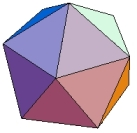HOME MATH DICTIONARY DOWNLOAD FEEDBACK DISCLAIMER
 Question: What is a Icosahedron ? Answer: An icosahedron is a polyhedron with 20 faces. A regular icosahedron has triangular faces each of which is an equilateral triangle. The word icos comes from the Greek for twenty. In geometry, an icosahedron is any polyhedron having 20 faces, but usually a regular icosahedron is implied, which has equilateral triangles as faces. The regular icosahedron is one of the five Platonic solids. It is a convex regular polyhedron composed of twenty triangular faces, with five meeting at each of the twelve vertices. It has 30 edges and 12 vertices. Its dual polyhedron is the dodecahedron.Example of an Acute Angled Triangle: